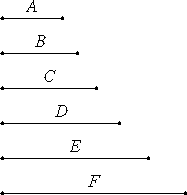Proposition 8

If as many numbers as we please beginning from a unit are in continued proportion, then the third from the unit is square as are also those which successively leave out one, the fourth is cubic as are also all those which leave out two, and the seventh is at once cubic and square are also those which leave out five.

Let there be as many numbers as we please, A, B, C, D, E, and F, beginning from a unit and in continued proportion.I say that B, the third from the unit, is square as are all those which leave out one; C, the fourth, is cubic as are all those which leave out two; and F, the seventh, is at once cubic and square as are all those which leave out five.

VII.Def.20

Since the unit is to A as A is to B, therefore the unit measures the number A the same number of times that A measures B. But the unit measures the number A according to the units in it, therefore A also measures B according to the units in A.

VIII.22

Therefore A multiplied by itself makes B, therefore B is square. And, since B, C, and D are in continued proportion, and B is square, therefore D is also square. For the same reason F is also square.

Similarly we can prove that all those which leave out one are square.

I say next that C, the fourth from the unit, is cubic are also all those which leave out two.

Since the unit is to A as B is to C, therefore the unit measures the number A the same number of times that B measures C. But the unit measures the number A according to the units in A, therefore B also measures C according to the units in A. Therefore A multiplied by B makes C. Since then A multiplied by itself makes B, and multiplied by B makes C, therefore C is cubic.

VIII.23

And, since C, D, E, and F are in continued proportion, and C is cubic, therefore F is also cubic. But it was also proved square, therefore the seventh from the unit is both cubic and square. Similarly we can prove that all the numbers which leave out five are also both cubic and square.

Therefore, if as many numbers as we please beginning from a unit are in continued proportion, then the third from the unit is square as are also those which successively leave out one, the fourth is cubic as are also all those which leave out two, and the seventh is at once cubic and square are also those which leave out five.

Q.E.D.

Guide

It’s unfortunate that Euclid didn't have the concept or notation for exponentiation.

In the continued proportion

1, a, a2, a3, a4, a5, a6, a7, etc.,

every second, a2, a4, a6, a8, etc., is a square;

every third, a3, a6, a9, a12, etc., is a cube; and

every sixth, a6, a12, a18, a24, etc., is both a square and a cube.

Use of this proposition

This proposition is used in four of the next five propositions.